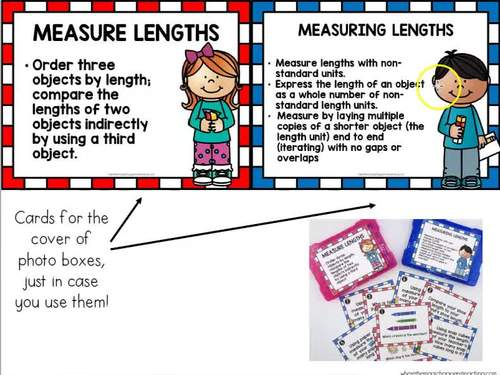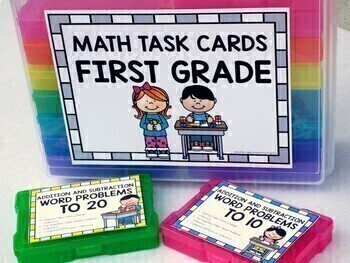Subject
Resource Type
File Type
Zip (47 MB|500+)
Standards
\$31.00
Bundle
List Price:
\$62.00
You Save:
\$31.00
Products in this Bundle (18)

showing 1-5 of 18 products

• Bundle Description
• Standards

This is a bundle with differentiated and editable math task cards for every single standard to be taught in 1st grade.

All the task cards in this bundle could be used for math centers, small groups, play seat scoot, and even whole group.

In this bundle, you will find 18 different sets of task cards that could be a perfect supplement to your BUILD math centers.

Please review the contents and the release of the upcoming task cards:

The price of this growing bundle will increase, as I add the task cards set.

Thank you so much for your purchase!

Laura G.♥

Permission to copy for single classroom use only.

Electronic distribution limited to single classroom use only.

Not for public display.

☻☻☻☻☻☻☻☻☻☻☻☻☻☻☻☻☻☻☻☻☻☻☻☻☻☻☻☻☻☻☻☻☻☻☻

Let’s Connect!!

Instagram

Pinterest

My Blog

Partition circles and rectangles into two and four equal shares, describe the shares using the words halves, fourths, and quarters, and use the phrases half of, fourth of, and quarter of. Describe the whole as two of, or four of the shares. Understand for these examples that decomposing into more equal shares creates smaller shares.
Compose two-dimensional shapes (rectangles, squares, trapezoids, triangles, half-circles, and quarter-circles) or three-dimensional shapes (cubes, right rectangular prisms, right circular cones, and right circular cylinders) to create a composite shape, and compose new shapes from the composite shape.
Distinguish between defining attributes (e.g., triangles are closed and three-sided) versus non-defining attributes (e.g., color, orientation, overall size); build and draw shapes to possess defining attributes.
Determine the unknown whole number in an addition or subtraction equation relating three whole numbers. For example, determine the unknown number that makes the equation true in each of the equations 8 + ? = 11, 5 = ▯ - 3, 6 + 6 = ▯.
Understand the meaning of the equal sign, and determine if equations involving addition and subtraction are true or false. For example, which of the following equations are true and which are false? 6 = 6, 7 = 8 - 1, 5 + 2 = 2 + 5, 4 + 1 = 5 + 2.
Total Pages
500+